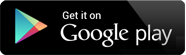## Video Lectures

Chapter 1 : Introduction to algorithms
Topic : Introduction to algorithms - What is algorithm, analysis of algorithm, Types of complexity, Running time analysis, How to Compare Algorithms, Rate of Growth, Types of Analysis, Asymptotic Notation, Big-O Notation, Omega-Ω Notation, Theta-�? Notation, Asymptotic Analysis, Performance characteristics of algorithms,
Content : Explanation 140 MB ,1:25:19 MIN , THEORY

Chapter 2 : Introduction to Data Structures
Topic : Introduction to Data Structures What is data structure, types,
Content : Data and Information, Data Structure, Classification of Data Structures, Primitive Data Types, Abstract Data Types, Data structure vs. File Organization, Operations on Data Structure, 66 MB ,34:48 MIN , THEORY

Chapter 2 : Introduction to Data Structures
Topic : Introduction to Array(1-d & 2-d),
Content : Introduction, One Dimensional Array, Memory Representation of One Dimensional Array, Traversing, Insertion, Deletion, Searching, Sorting, Merging of Arrays, Multidimensional Arrays, Memory Representation of Two Dimensional Arrays, 52 MB ,31:36 MIN , THEORY + PRACTICAL

Chapter 2 : Introduction to Data Structures
Topic : Stack Applications Matching Parenthesis,
Content : Matching Parenthesis, 9 MB ,5:29 MIN , THEORY + PRACTICAL

Chapter 2 : Introduction to Data Structures
Topic : List data structures
Content : Two way Linked List, Traversing a Two way Linked List, Searching in a Two way linked List, Insertion of an element in Two way Linked List, Deleting a node from Two way Linked List, 59 MB ,30:28 MIN , THEORY + PRACTICAL

Chapter 2 : Introduction to Data Structures
Topic : List data structures
Content : Linked List, One-way Linked List, Traversal of Linked List, Searching, Memory Allocation and De-allocation, Insertion in Linked List, Deletion from Linked List, 80 MB ,44:53 MIN , THEORY + PRACTICAL

Chapter 2 : Introduction to Data Structures
Topic : Applications of Stack, Evaluation of Arithmetic Expression,
Content : Explanation 48 MB ,25:55 MIN , THEORY + PRACTICAL

Chapter 2 : Introduction to Data Structures
Topic : List data structures Applications of the Linked list, Representation of Polynomials, Storage of Sparse Arrays, Implementing other Data Structures.
Content : Explanation 30 MB ,16:35 MIN , THEORY + PRACTICAL

Chapter 2 : Introduction to Data Structures
Topic : List data structures
Content : One-way Linked List, Traversal of Linked List, Searching, Memory Allocation and De-allocation, Insertion in Linked List, Deletion from Linked List, 97 MB ,51:5 MIN , THEORY + PRACTICAL

Chapter 2 : Introduction to Data Structures
Topic : Stacks Introduction, Operations on the Stack Memory Representation of Stack, Array Representation of Stack, Applications of Stack,
Content : Explanation 71 MB ,41:40 MIN , THEORY + PRACTICAL

Chapter 1 : Introduction to algorithms
Topic : Estimating running time, number of steps of executions on paper, Idea of Computability
Content : Explanation 29 MB ,14:54 MIN , THEORY

Chapter 1 : Recursion
Topic : Recursion - What is recursion, Recursion vs Iteration, recursion applications like Factorial of a number
Content : Explanation 19 MB ,10:55 MIN , THEORY + PRACTICAL

Chapter 2 : Basic Sorting Techniques
Topic : Basic Sorting Techniques - Insertion Sort
Content : Explanation 96 MB ,56:6 MIN , THEORY + PRACTICAL

Chapter 2 : Basic Sorting Techniques
Topic : Basic Sorting Techniques - Bubble Sort
Content : Explanation 111 MB ,1:4:23 MIN , THEORY + PRACTICAL

Chapter 4 : Selection Techniques
Topic : Selection Techniques - Selection by Sorting, Partition-based Selection Algorithm, Finding the Kth Smallest Elements in Sorted Order
Content : Explanation 63 MB ,35:38 MIN , THEORY + PRACTICAL

Chapter 2 : Basic Sorting Techniques
Topic : Basic Sorting Techniques - Selection Sort
Content : Explanation 128 MB ,1:13:25 MIN , THEORY + PRACTICAL

Chapter 1 : Recursion
Topic : Fibonacci series & their comparative analysis with respect to iterative version
Content : Explanation 27 MB ,14:55 MIN , THEORY

Chapter 1 : Recursion
Topic : Tower of hanoi problem
Content : Explanation 12 MB ,7:28 MIN , THEORY + PRACTICAL

Chapter 5 : String Algorithms
Topic : String Algorithms - Pattern matching in strings, Brute Force Method & their comparative analysis
Content : Explanation 40 MB ,22:32 MIN , THEORY

Chapter 1 : Algorithm Design Techniques
Topic : Introduction to various types of classifications/design criteria and design techniques
Content : Explanation 78 MB ,43:40 MIN , THEORY

Chapter 3 : Divide-n-Conquer
Content : Explanation 64 MB ,37:58 MIN , THEORY

Chapter 2 : Greedy Technique
Topic : Greedy Technique - Concept, Advantages & Disadvantages, Applications, Implementation using problems like - file merging problem using Huffman Algorithm
Content : Explanation 164 MB ,1:35:47 MIN , THEORY

Chapter 3 : Divide-n-Conquer
Topic : Divide-n-Conquer Implementation using problems like - merge sort
Content : Merge Sort Explanation 82 MB ,44:36 MIN , THEORY + PRACTICAL

Chapter 4 : Dynamic Programming
Topic : Dynamic Programming - Concept, Advantages & Disadvantages, Applications, Implementation using problems like - Fibonacci series, Longest Common subsequence
Content : Explanation 106 MB ,1:4:8 MIN , THEORY + PRACTICAL

Chapter 2 : Greedy Technique
Topic : Greedy Technique File Merging Problem
Content : Greedy Technique File Merging Problem 15 MB ,8:19 MIN , THEORY

Chapter 3 : Divide-n-Conquer
Topic : Divide-n-Conquer - Strassen's Matrix Multiplication
Content : Strassen's Matrix Multiplication 26 MB ,13:53 MIN , THEORY

Chapter 4 : Dynamic Programming
Topic : Dynamic Programming Implementation using problems like Factorial of a number
Content : problems like Factorial of a number 10 MB ,6:13 MIN , THEORY

Chapter 5 : Backtracking Programming
Topic : Backtracking Programming - Concept, Advantages & Disadvantages, Applications, Implementation using problems like N-Queen Problem
Content : Explanation 35 MB ,19:43 MIN , THEORY

## The Shikshak App

The Shikshak App is an honest attempt to provide quality education.